To Reverse a doubly linked list we have two approaches either reverse the links of every node or either reverse the values of nodes. in this tutorial, we are going to reverse a doubly-linked list by reversing their links.

like if we have a doubly-linked list that has four nodes. as you see in the image given below.after reversing this linked list the start variable refers to the last node of the list and the first node becomes the last node and the last node becomes the first node. so our new reversed linked list should be like this as you see in the image given below.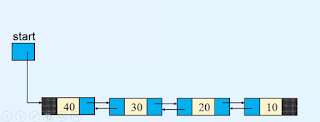## Method to Reversing a Doubly linked list

so to reverse a linked list first we need two references p1 and p2. initially, we set p1 pointing to the first node of the linked list and p2 pointing to the second node of the linked list.after that, we store the p1 node's previous link part into the p1 node's next link part. as you see in the given below image.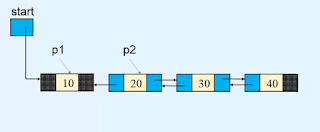and then we store the p2 node's reference into the p1 node's previous link part.after that, we start a loop and that will be run till the value of variable p2 does become None or Null. it means the loop will run until we reached the last node of the linked list.

in every iteration first, we store the p2 node's next link part's value into the p2 node's previous link part.second, we store the p1 node's reference into p2 node's next link part.third, we set p1 to the next node that comes after the node p1 using the p2 node.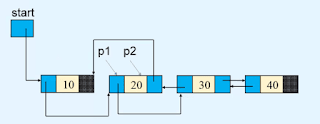fourth we set p2 to the next node that comes after the node p2 using the previous link part of node p2.so in every iteration, these four conditions will apply and this condition will run till we don't reach the last node of the linked list or till the value of the node, p2 becomes None or Null.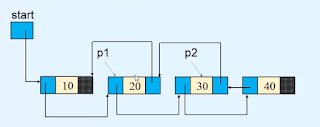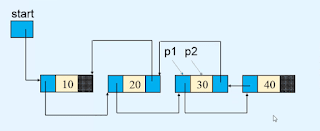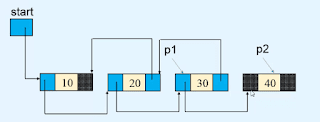so now the value of variable p2 becomes None means we reached the last node of the linked list so we store the p1 node's reference into the start variable because the start variable always refers to the first node of the linked list.#### Python program for reversing a doubly linked list.

```class Node(object):

def __init__(self, value):
self.info = value
self.prev = None        self.next = None

def __init__(self):
self.start = None
def display_list(self):
if self.start is None:
print("LIst is empty")
return
print("List is : ")
p = self.start
while p is not None:
print(p.info, " ", end='')
p = p.next
print()

def insert_in_beginning(self, data):
temp = None(data)
temp.next = self.start
self.start.prev = temp
self.start = temp

def insert_in_empty_list(self, data):
temp = Node(data)
self.start = temp

def insert_at_end(self, data):
temp = Node(data)
p = self.start
while p.next is not None:
p = p.next
p.next = temp
temp.prev = p

def create_list(self):
n = int(input("Enter the number of nodes : "))
if n == 0:
return        data = int(input("Enter the first element to be inserted : "))
self.insert_in_empty_list(data)

for i in range(n - 1):
data = int(input("Enter the next element to be inserted : "))
self.insert_at_end(data)

def insert_after(self, data, x):
temp = Node(data)
p = self.start
while p is not None:
if p.info == x:
break            p = p.next

if p is None:
print(x, " not present in the list")
else:
temp.prev = p
temp.next = p.next
if p.next is not None:
p.next.prev = temp
p.next = temp

def insert_before(self, data, x):
if self.start is None:
print("List is empty")
return
if self.start.info == x:
temp = Node(data)
temp.next = self.start
self.start.prev = temp
self.start = temp
return
p = self.start
while p is not None:
if p.info == x:
break            p = p.next

if p is None:
print(x, " not present in the list")
else:
temp = Node(data)
temp.prev = p.prev
temp.next = p
p.prev.next = temp
p.prev = temp

def delete_first_node(self):
if self.start is None:
return        if self.start.next is None:
self.start = None            return        self.start = self.start.next
self.start.prev = None
def delete_last_node(self):
if self.start is None:
return        if self.start.next is None:
self.start = None            return
p = self.start
while p.next != None:
p = p.next
p.prev.next = None
def delete_node(self, x):
if self.start is None:
return        if self.start.next is None:
if self.start.info == x:
self.start = None            else:
return
if self.start.info == x:
self.start = self.start.next
self.start.prev = None            return
p = self.start.next
while p.next is not None:
if p.info == x:
break
p = p.next

if p.next is not None:
p.prev.next = p.next
p.next.prev = p.prev
else:
if p.info == x:
p.prev.next
else:

def reverse_list(self):
if self.start is None:
return
p1 = self.start
p2 = p1.next
p1.next = None        p1.prev = p2
while p2 is not None:
p2.prev = p2.next
p2.next = p1
p1 = p2
p2 = p2.prev
self.start = p1

#################################################################3

list.create_list()

while True:
print("1. Display list")
print("2. Insert in empty list")
print("3. Insert a node in the beginning of the list")
print("4. Insert a node at the end of the list")
print("5. Insert a node after a specified node")
print("6. Insert a node before a specified node")
print("7. Delete first node")
print("8. Delete last node")
print("9. Delete any node")
print("10. Reverse the list")
print("11. Quit")

option = int(input("Enter your choice : "))

if option == 1:
list.display_list()
elif option == 2:
data = int(input("Enter the element to be inserted : "))
list.insert_in_empty_list(data)
elif option == 3:
data = int(input("Enter the element to be inserted : "))
list.insert_in_beginning(data)
elif option == 4:
data = int(input("Enter the element to be inserted : "))
list.insert_at_end(data)
elif option == 5:
data = int(input("Enter the element to be inserted : "))
x = int(input("Enter the element after which to insert : "))
list.insert_after(data, x)
elif option == 6:
data = int(input("Enter the element to be inserted : "))
x = int(input("Enter the element before which to inserted : "))
list.insert_before(data, x)
elif option == 7:
list.delete_first_node()
elif option == 8:
list.delete_last_node()
elif option == 9:
data = int(input("Enter the element to be deleted : "))
list.delete_node(data)
elif option == 10:
list.reverse_list()
elif option == 11:
break    else:
print("Wrong option")
print()```